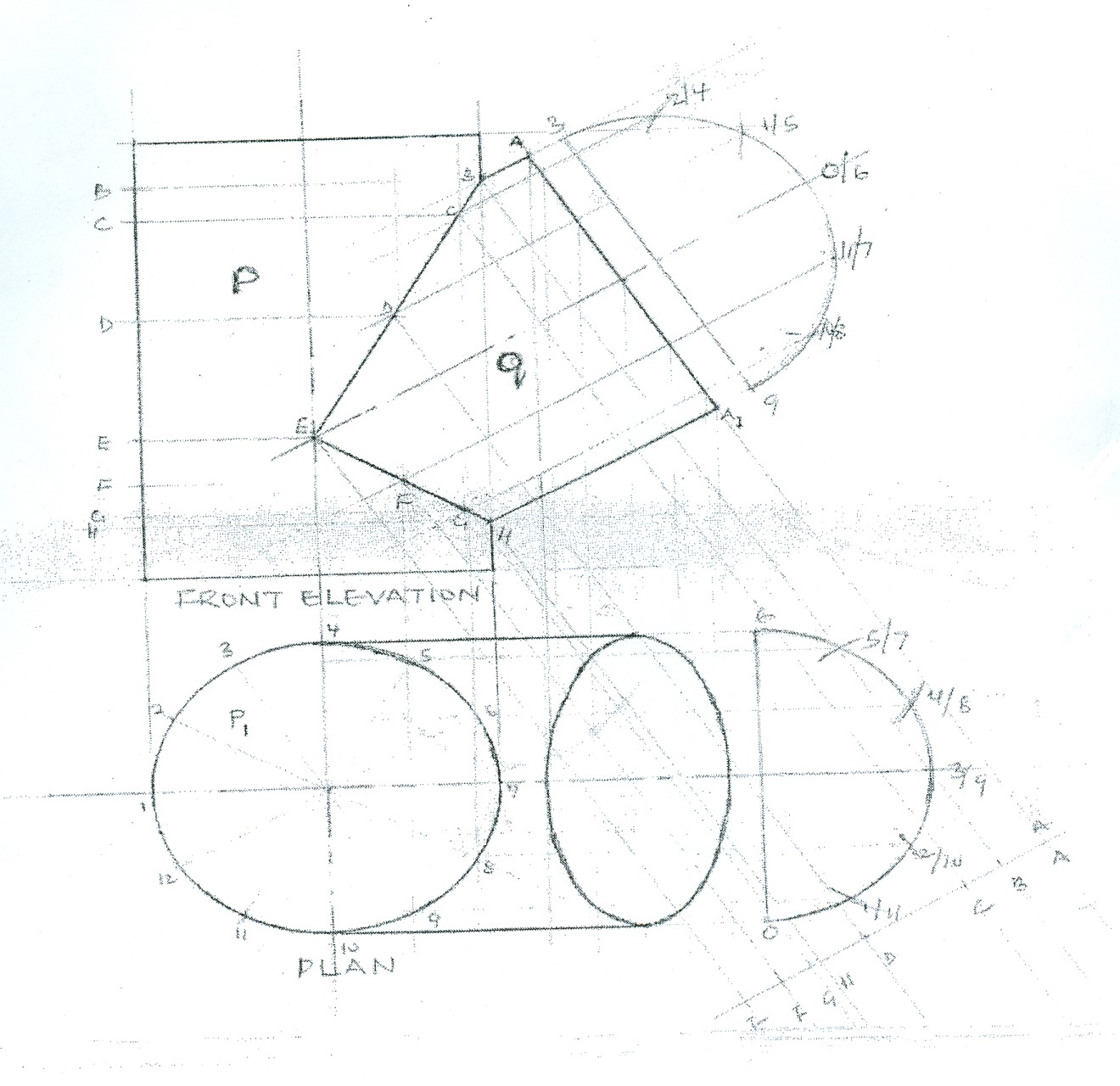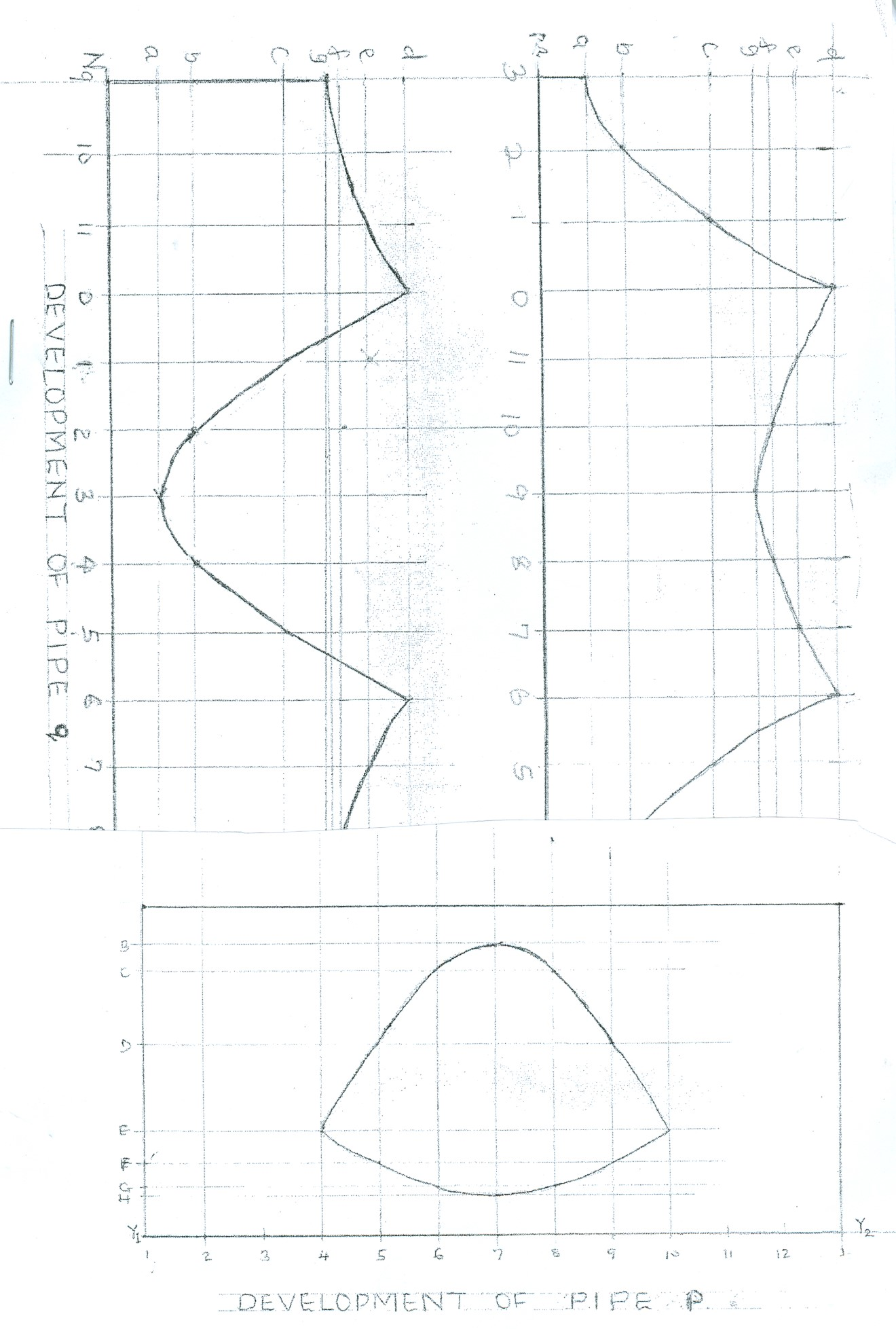Technical Drawing Paper 2, Nov/Dec. 2014
 Questions: 1 2 3 4 Main
Weakness/Remedies
Strength

Question 1

(1)       Two equal pipes of diameter 60 mm intersect at 30o as shown above.  Draw, full size, the:

• given view with the line of intersection;
• complete plan;
• development of pipe.
_____________________________________________________________________________________________________
Observation

This was a question on curve of intersection, candidates were required to draw, full size, the given view with the line of intersection; complete plan; development of pipe.  Most candidates attempted this question but only a few got it right.

Candidates were able to copy the given view, draw the plan and the complete the line of intersection of the elevation correctly.

However, they were unable to complete the plan and the development of the pipe P or pipe Q.

Candidates were expected to do the following:

DRAWING LINE OF INTERSECTION

A.         COPYING THE GIVEN VIEW

1. draw the entre lines;
2. draw the vertical pipe P;
3. draw the intersecting pipe Q at 30O.

B.         DRAWING THE PLAN

1. project down from the elevation to draw the plan;
2. draw the base circle for pipe P and divide into 12 equal parts;
3. draw tangential lines for pipe Q.

C.        COMPLETING LINE OF INTERSECTION

ELEVATION

1. draw circle/semi-circle on pipe Q and divide into 12/6 equal parts;
2. project lines from divisional points on circumference parallel to the sides of pipe Q;
3. draw circle/semi-circle on pipe Q on plan and divide it into 12/6 equal parts;
4. draw horizontal lines from the divisional points on circumference on pipe Q to intersect the corresponding points on pipe P in the plan;
5. project lines from the divisional points on the circumference of pipe P in the plan to intersect the corresponding lines from pipe Q on elevation;
6. draw curve of intersection for pipe P.

COMPLETING THE PLAN

1. project down from points on end AA1 of pipe Q on the elevation to intersect the corresponding lines from the circle/semi-circle on pipe Q on plan.
2. Locate and draw the face of pipe Q on the plan.

D.        DEVELOPMENT OF PIPE P OR PIPE Q

1. draw the circumference of pipe P or pipe Q (equal diameters)

using the circle P1 on plan and divide it into 12 equal parts;

1. draw vertical lines from points 4 to point 10 on line Y1Y2 or from points 3, 2, 1, 0, 11 to 3 on line MR;
2. transfer vertical co-ordinates B, C, D to H on pipe P or d, e, f, g to a on pipe Q from the curve of intersection on the development;
3. Locate and complete the development of pipe P or Q.

The solution is shown below: